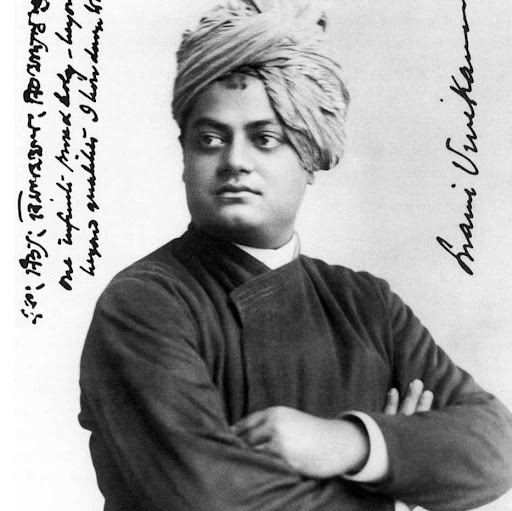3:32 AM

6 hours later…9:34 AM
say intuitively why water expands when temperature drops?9:53 AM
@user863565 There is no intuition because this is highly anomalous behaviour compared to other liquids, but see physics.stackexchange.com/a/29284/50583.I have my hypothesis for this i will try to experiment before saying anything stupid 😅10:49 AM
@ACuriousMind All conductor are polar molécules right?many conductors are metals and hence not molecules at all, but more crystal structures (lattices) of atoms11:26 AM
Very simply question
why is $dp^\mu dp_\mu = d^4p$? Shouldn't it be $=dp^1dp_1 + ...$I don't know what $\mathrm{d}p^\mu\mathrm{d}p_\mu$ is supposed to be
differentials exist only with upper indices, you can't lower their index, there is no such thing as $\mathrm{d}p_\mu$Mhm yeah that's what I thought
1To prove $\frac{\mathrm{d}^3\vec{p}}{E}$ is Lorentz invariant is to prove $$\frac{\mathrm{d}^3\vec{p}}{E} = \frac{\mathrm{d}^3\vec{p}'}{E'} \qquad(\mathrm{d}^3\vec{p} := \mathrm{d}p_x \mathrm{d}p_y \mathrm{d}p_z). \tag{1}$$ Suppose reference system $\Sigma'$ is moving at speed $v=\beta$ ($c=1$)...

See the first line of this answer
Why is $d^4p$ so obviously invariantt?I have no idea what the expression $\mathrm{d}p^\mu\mathrm{d}p_\mu$ is supposed to mean, but $\mathrm{d}^4p$ is invariant simply because it's a volume element and so it transforms under linear transformations $p\mapsto \Lambda p$ by a factor of $\mathrm{det}(\Lambda)$, and the determinant of Lorentz transformations connected to the identity is 1.okay cool
is $(p_0)^2$ lorentz invariant?no11:35 AM
Mhm. Could you explain this part of a proof in Tongs notes
Particularly the "but the choice of branch is another Lorentz..."
And how you 'put it together'@JakeRose A Lorentz transformation connected to the identity cannot change the branch of solutions to $p^2 = m^2$ a vector lies on, because the condition $p^2 = m^2$ is invariant and so every transformation of $p$ still needs to lie on one of the branches
that is, a vector and its transformation need to be continuously connected via a path that lies on the hyperboloids cut out by $p^2 = m^2$, and this is cannot be for vectors on different branchesI'm. not sure I followI discuss this almost in the same words here

2 hours later…1:38 PM
$X_2=\big\{(x,y)\mapsto\big(ax,\frac{y}{a^2}\big)\big\}.$ Does this transformation have a name? Is it related to a Lorentz boost?

1 hour later…2:44 PM
Uh! ACM why do u use such horrific images as your dp?@ACuriousMind
Very very long time.............3:15 PM
the picture expresses my state of mind very well, I'm not sure what problem you have with it :P

4 hours later…7:00 PM
This from Math.SE could have been asked on Physics.SE. I tried to answer it the best I could:
1According to the answer given here, the diagonal generalized Gell-Mann matrices are not unique. But what exactly is meant by this? Are they just saying that we can multiply the diagonal matrices by a constant and still have a valid generator of the group since the matrices will still be orthogo...